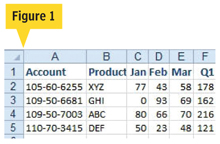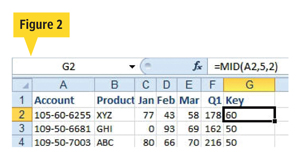Technology

How to isolate data in Microsoft Excel.

USER PROBLEM: My company assigns an account ID in the format SSS-XX-YYYY. I need to isolate the XX portion of the account ID in order to subtotal or sort the data. What is the best way to do that?SOLUTION: You can insert a new column and use the MID function to isolate the necessary digits from the Account field (see Figure 1).

The MID function takes three arguments: (1) a cell that contains a text value, (2) the character number where you want the result to start, and (3) the length of the result.

In a well-formed account number, such as 123-45-6789, you can predict that the start of the second segment will always be in the fifth character position and the length of the second segment is always two characters. Therefore, you can follow these steps:1. In a blank column, such as column G, enter a heading such as Key.

2. In cell G2, enter the formula =MID(A2,5,2).

3. Copy the formula down to all rows (see Figure 2).

ADDITIONAL DETAILS: To capture the final four digits of the account number, you could either use =MID(A2,8,4) or =RIGHT(A2,4). In that way, you can sort by the new column and add subtotals based on the Account field.

If you would like to submit a question to Bill “MrExcel” Jelen, e-mail him at [email protected].